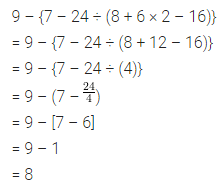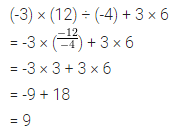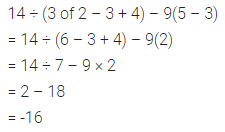# ML Aggarwal Class 7 Solutions for ICSE Maths Chapter 1 Integers Ex 1.5

## ML Aggarwal Class 7 Solutions for ICSE Maths Chapter 1 Integers Ex 1.5

Question 1.
7 – 8 ÷ (-2) + 3 × (-4)
Solution:Question 2.
9 – {7 – 24 ÷ (8 + 6 × 2 – 16)}
Solution:Question 3.
-11 – [-6 – {3 – 5(8 ÷ 4 – 1)}]
Solution:Question 4.
(-3) × (12) ÷ (-4) + 3 × 6
Solution:Question 5.
14 ÷ (3 of 2 – 3 + 4) – 9(5 – 3)
Solution: